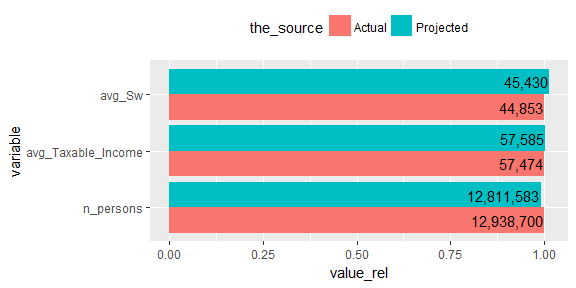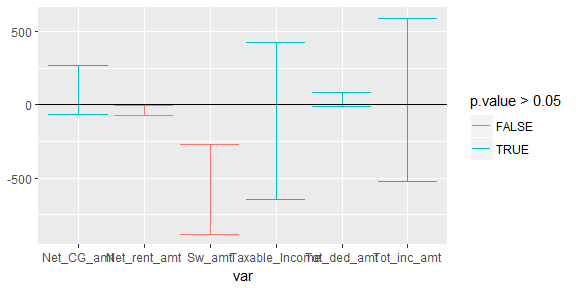# Performance of project

## How well does the project function work one year in advance?

library(knitr)
library(data.table)
if (requireNamespace("taxstats", quietly = TRUE)){
library(taxstats)
sample_files_all <- get_sample_files_all()
} else {
install.packages("taxstats", repos = "https://hughparsonage.github.io/drat/", type = "source")
library(taxstats)
sample_files_all <- get_sample_files_all()
}
library(grattan)
library(dtplyr)
library(dplyr)
library(magrittr)
library(ggplot2)
library(scales)
library(broom)
sample_file_1314_projected <-
sample_file_1213 %>%
copy %>%
mutate(WEIGHT = 50) %>%
project(h = 1L, fy.year.of.sample.file = "2012-13", .recalculate.inflators = TRUE) %>% .[]

This should be pretty damn close!

data.table(the_source = c("Actual", "Projected"),
n_persons = c(nrow(sample_file_1314) * 50, sum(sample_file_1314_projected$WEIGHT)), avg_Taxable_Income = c(mean(sample_file_1314$Taxable_Income), mean(sample_file_1314_projected$Taxable_Income)), avg_Sw = c(mean(sample_file_1314$Sw_amt), mean(sample_file_1314_projected\$Sw_amt))
) %>%
melt.data.table(id.vars = "the_source") %>%
group_by(variable) %>%
mutate(value_rel = value / first(value)) %>%
# dcast.data.table(variable ~ the_source) %>%
ggplot(aes(x = variable, y = value_rel, fill = the_source)) +
geom_bar(stat = "identity", position = "dodge") +
geom_text(aes(label = comma(round(value))), position = position_dodge(width = 0.9), hjust = 1.02) +
coord_flip() +
theme(legend.position = "top")conf_int_of_t.test <- function(variable){
t_test <- t.test(sample_file_1314[[variable]], sample_file_1314_projected[[variable]])
t_test %>%
tidy(.) %>%
mutate(var = variable) %>%
as.data.table
}

lapply(c("Sw_amt", "Net_rent_amt", "Net_CG_amt", "Tot_inc_amt", "Tot_ded_amt", "Taxable_Income"),
conf_int_of_t.test) %>%
rbindlist %>%
select(var, conf.low, conf.high, p.value) %>%
ggplot(aes(x = var, ymin = conf.low, ymax = conf.high, color = p.value > 0.05)) +
geom_errorbar() +
geom_hline(yintercept = 0)## Years not in sample files

Other tests can be found in the tests/ folder to compare the tax collections in those years.